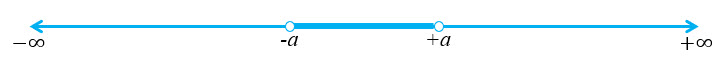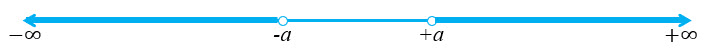Inequation: A statement involving variable(s) and the sign of inequality that is,$>, < , \geq \text{ or } \leq$ is called an inequation or an inequality. Some examples of linear inequations are$9x-15>0,5x- 4 \geq 0, 3x+2<0$$2x+3y \leq 6, 3x-2y \geq 12, x+y<4,2x+y \geq 6$

Linear Inequation in One Variable: Let$a$ be a non-zero real number and$x$ be a variable. Then inequations of the form$ax +b <0, ax +b >0, ax +b \geq$ and$ax+b \leq 0$ are known as linear inequations in one variable$x$.

For example,$9x-15>0,5x- 4 \geq 0, 3x+2<0$ and$2x-3 \leq 0$ are linear inequations in one variable.

Linear Inequation in Two Variable: Let$a, b$ be non-zero real numbers and$x, y$ be variables. Then inequations of the form$ax+by < c, ax+by \leq c, ax+by > c$ and$ax+by \geq c$ are known as linear inequations in two variables$x$ and$y$.

For example,$2x+3y \leq 6, 3x-2y \geq 12, x+y<4,2x+y \geq 6$ are linear inequations in two variables$x$ and$y$.

Quadratic Inequation: Let a be a non-zero real number. Then an inequation of the form$ax^2 +bx+ c < 0$, or$ax^2 +bx + c \leq 0$, or$ax^2 +bx+ c> 0$, or$ax^2 +bx + c \geq 0$ is known as a quadratic inequation.

For example,$x^2 +x-6 < 0, x^2 - 3x + 3 \geq 0, 2x^2 + 3x + 1 > 0$ and$x^2 - 5x + 4 \leq 0$ are quadratic inequations.

Solving linear inequations in one variable: ln the process of solving an inequation, we use mathematical simplifications which are governed by the following rules:

• Same number may be added to (or subtracted from) both sides of an inequation without changing the sign of inequality.
• Both sides of an inequation can be multiplied (or divided) by the same positive real number without changing the sign of inequality . However, the sign of inequality is reversed when both sides of an inequation are multiplied or divided by a negative number.
• Any term of an inequation may be taken to the other side with its sign changed without affecting the sign of inequality.

How to solve a linear inequation?

1. Obtain the linear inequation.
2. Collect all terms involving the variable on one side of the inequation and  the constant terms on the other side.
3. Simplify both sides of inequality in their simplest forms to reduce the inequation in the form$ax < b \text{ or } ax \leq b \text{ or } ax > b \text{ or } ax \geq b$
4. Solve the inequation obtained in step 3 by dividing both sides of the inequation by the coefficient of the variable.
5. Write the solution set obtained in step IV in the form of an interval on the real line.

Inequations involving modulus of the variable

Case 1: If$a$ is a positive real number, then

i)$|x| < a \Leftrightarrow -a < x < a \text{ i.e } a \in ( -a, a)$ii)$|x|\leq a \Leftrightarrow -a \leq x \leq a \text{ i.e } a \in [ -a, a ]$Case 2: If$a$ is a positive real number, then

i)$|x| > a \Leftrightarrow x < -a \text{ or } x > a$ii)$|x| \geq a \Leftrightarrow x \leq -a \text{ or } x \geq a$Case 3: Let$r$ be a positive real number and$a$ be a fixed real number. Then,

i)$|x - a| < r \Leftrightarrow a-r < x < a+r \text{ i.e } x \in ( a-r, a+r)$

ii)$|x - a| \leq r \Leftrightarrow a-r \leq x \leq a+r \text{ i.e } x \in [ a-r, a+r ]$

iii)$|x -a| > r \Leftrightarrow x < a-r \text{ or } x > a+r$

iv)$|x -a| \geq r \Leftrightarrow x \leq a-r \text{ or } x \geq a+r$

Case 4: Let$a, b$ be positive real numbers. Then

i)$a < |x| < b \Leftrightarrow x \in (-b, -a) \cup (a, b)$

ii)$a \leq |x| \leq b \Leftrightarrow x \in [-b, -a] \cup [a, b]$

iii)$a < |x-c| < b \Leftrightarrow x \in (-b+c, -a+c) \cup (a+c, b+c)$

iv)$a \leq |x-c| \leq b \Leftrightarrow x \in [-b+c, -a+c] \cup [a+c, b+c]$

Graphical solution of linear inequations in two variables:

1. Convert the given inequations, say$ax + by < c$, into the equation$ax + by = c$ which represents a straight line in$xy-$plane.
2. Put$y = 0$ in the equation obtained in step 1 to get the point where the line meets with x-axis. Similarly, put$x = 0$ to obtain a point where the line meets with y-axis.
3. Join the points obtained in step 2 to obtain the graph of the line obtained from the given inequation. In case of a strict inequality i.e.$ax +by or$ax +by > c$, draw the dotted line otherwise mark it thick line.
4. Choose a point, if possible$(0, 0)$, not lying on this line : Substitute its coordinates  in the inequations. If the inequation is satisfied,  then shade the portion of the plane which contains the point. Otherwise, shade the portion of the plane that does not contain this point.
5. Repeat step 4 for the other inequation also.
6. The common shaded area obtained is the solution set for the two inequations.
7. In case of the inequalities$ax +by \leq c$ and$ax +by \geq c$, the points on the line are also part of the shaded region. While, in case of the inequalities$ax +by < c$ and$ax +by > c$, the points on the line are also not part of the shaded region.